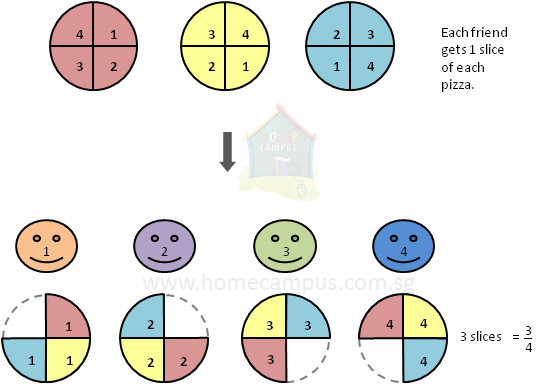## Concept of Fraction as a Division

Practice Unlimited Questions

#### 2. Show that 3 divided by 4 is 3 4 .

Let's suppose we have 3 pizzas that are divided equally among 4 friends  =>  3  ÷  4.
We will show that each friend gets  =>
 3 4
of a pizza.3  ÷  4
 1 4
of   3 (pizzas)
 1 4
×  3
 1 4
×
 3 1

 1 x 3 4 x 1

 3 4
3 pizzas are divided among 4 friends.

So, each of the friend gets
 1 4
of each of the 3 pizzas.
Therefore, each friend gets
 3 4
of the pizza.